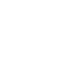the-marks-obtained-out-of-20-by-30-students-of-class-in-a-test-are-1Top matches
Q1.
The Marks obtained ( out of 20) by 30 students of class in a test are as follows :
14,16,15,11,15,14,13,16,8,10,7,11,18,15,14,19,20,7,10,13,12,14,15,13,16,17,14,11,10,20
Prepare a histogram from the frequency distribution table obatained in question.
Q2.

Marks obtained by students out of 20 in a class test are written on the board. What is the range of the marks?

12 ,14 , (-1), (-6), 15, 0, 8, 2.  [2 MARKS]

Q3.
The marks of 30 students of a class, obtained in a test (out of 75), are given below:
42,21,50,37,42,37,38,42,49,52,38,53,57,47,29
59,61,33,17,17,39,44,42,39,14,7,27,19,54,51.
Form a frequency table with equal class intervals.
Q4.
In a class of 40 students, marks obtained by the students in a class test (out of 10) are given below.
 Marks 1 2 3 4 5 6 7 8 9 10 Number of students 1 2 3 3 6 10 5 4 3 3
Calculate the following for the given distribution:
Mode
Q5.
In a class of 40 students, marks obtained by the students in a class test (out of 10) are given below.
 Marks 1 2 3 4 5 6 7 8 9 10 Number of students 1 2 3 3 6 10 5 4 3 3
Calculate the following for the given distribution:
Median